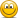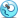# Monotonicity

#### Sixcarbs

##### Active Member
Subscriber
Hi, this is not about specific question but about the concept of Monotonicity. It did not seem intuitive to me when I read it and I was hoping it was an error, but I just watched the video and it is the same.

IF Y>=X then rho(Y) <= rho (X)

If Y returns more than X, than the risk of Y must be less than the risk of X.

Why would anyone invest in X if they can do better with Y for less risk? It just seems to counter everything we have been discussing.

Thanks

Further thoughts: Or does this mean that the VaR(Y) has to be less than or equal to the VaR(X)? Even so, why ever invest in X?

Last edited:

#### Detective

##### Active Member
I think you are overthinking it. The context here is evaluating a risk measure, and not which portfolio should be invested in. rho(.) here is the risk measure being evaluated, it could be VaR or really any risk measure.

The intuition is if you have a portfolio (or risky asset) Y that has expected returns larger than portfolio (or risky asset) X in almost all scenarios, then intuitively you would expect the risk on portfolio Y to be less than portfolio X. This is not saying that you should invest in portfolio X; in fact, you've intentionally constructed a case where you can definitively argue Y is better than X.

Conceivably you would expect all risk measures to satisfy this property, but I can certainly construct a very dumb risk measure that fails to satisfy this. Assuming all risk measures satisfy monotonicity is not something we can take for granted, so it should be tested -- and it's one of the properties used to determine if a risk measure is coherent.

The major theme here as far FRM is considered, VaR is not coherent risk measure (as it doesn't necessarily satisfy subadditivity in all cases), whereas ES (Expected Shortfall) is a coherent risk measure.

#### David Harper CFA FRM

##### David Harper CFA FRM
Staff member
Subscriber
Hi @Sixcarbs and @Detective The Monotonicity property of coherence has been a challenge to interpret but I happen to agree with Detective's interpretation. Please note there is a thread over here at https://forum.bionicturtle.com/threads/coherent-risk-measure-monotonicity.8214/ where I propose that absolute normal VaR (but not relative VaR) illustrates this.

Per Dowd: Monotonicity: Y ≥ X --> ρ(Y) < ρ(X)

Say:
µ(Y) = +5% and σ(Y) = 10.0%
µ(X) = +3% and σ(Y) = 10.0%

99.0% aVaR(Y) = -5% + 10.0%*2.33 = 18.3%
99.0% aVaR(X) = -3% + 10.0%*2.33 = 20.3%

In this way, the expected future value of Y dominates X due to higher expected return, Y > X, such that is has lower risk per aVaR.
i.e., Y ≥ X per µ(Y) > µ(Y) and ρ(Y) < ρ(X) per 18.3% < 20.3%

I think this reconciles with Dowd's technical interpretation (emphasis mine):
Footnotes 11 and 12, page 33
11. At a deeper level, we can also start with the notion of an acceptance set, the set of all positions acceptable to some stakeholder (e.g., a financial regulator). We can then interpret the risk measure ρ(.) as the minimum extra cash that has to be added to the risky position and invested prudently in some reference asset to make the risky position acceptable. If ρ(.) is negative, its negativity can be interpreted as the maximum amount that can be safely withdrawn, and still leave the position acceptable.

12. The other conditions can be understood from the last footnote. Monotonicity means that a random cash flow or future value Y that is always greater than X should have a lower risk: this makes sense, because it means that less has to be added to Y than to X to make it acceptable, and the amount to be added is the risk measure. Positive homogeneity implies that the risk of a position is proportional to its scale or size, and makes sense if we are dealing with liquid positions in marketable instruments. Translational invariance requires that the addition of a sure amount reduces pari passu the cash needed to make our position acceptable, and is obviously valid when one appreciates that the cash needed is our risk measure

Last edited:

#### bollinez123

##### New Member
Hi David, I am not really able to connect this with the BIS 19 "Messages from the Academic Literature on Risk Measuring for the Trading Books", where it is written:

Monotonicity: R(L1) < R(L2) if L1 < L2

Could you please provide a little example how to interpret it in comparison to Dowd's version:

Monotonicity: Y ≥ X --> ρ(Y) < ρ(X)

Thank you a lot!

Plus: I just read through the thread https://forum.bionicturtle.com/threads/coherent-risk-measure-monotonicity.8214/ but somehow it confuses me a bit. As you are stating

Hey @ami44 I was just supporting a (lower board) source Q&A (same question as here: https://forum.bionicturtle.com/threads/l2-t5-71-coherent-risk-measures.3655/#post-33122) and @Aenny helpfully shared this paper, which I've never seen before: http://www.columbia.edu/~mh2078/RiskMeasures.pdf
This is interesting because it speaks exactly to our conversation. This author is using losses (L1 and L2) rather than expected future values (X and Y in Dowd), so as we confirmed ourselves, he's got an alternative definition for monotonicity that only looks different.

It's also interesting because his Translation Invariance matches yours above, your X is Loss: rho(X+c) = rho(X) + c.
Ergo, it looks like you are correct about that and I am incorrect ... although I don't understand how it can be, it still doesn't make sense to me that adding cash (or capital) can increase the risk. And, in this paper, greater rho is apparently greater risk. So I disagree with this paper, or probably I don't understand it. Because it agrees with you!The cash part is the part that actually confuses me. Does that mean the R(L1) < R(L2) if L1 < L2 part is indeed reasonable due to the cash argument? Maybe you can bring some light into the darknessLast edited:

#### bollinez123

##### New Member

Hi @Aenny

Thanks but I am following Dowd, who is assigned for coherence. The paper you reference uses loss (L) rather than gain (i.e., X and Y are returns or future cash flows or future values). In this way, they are equivalent with respect to Monotonicity:
• Y ≥ X --> ρ(Y) < ρ(X)
• L1 ≤ L2 --> ρ(L1) < ρ(L2); those are identical in my opinion, as X and Y are future values but L1 and L2 are future losses
These coherence criteria are firstly symbolic, the best way to approach them (in my mere opinion) is just intuitively. Intuitively, these criteria seem quite natural. It seems like too much attachment to the notation can lead to confusion (as usual in the FRM!). I've never seen your alternative formulas, but because I understand the intuition of monotonicity, it's not a problem ...

Also, I am actually a friend of thinking about everything as functions and equations and the following statement about the relationship helped me also understanding it a bit more (for those that are still struggling):

https://www.statisticshowto.datasciencecentral.com/monotonic-relationship/
"If an increase in the independent variable causes a decrease in the dependent variable (image b), this is called a monotonic inverse relationship. An inverse relationship is the same thing as a negative correlation. A monotonic direct relationship is where an increase in the independent variable causes an increase in the dependent variable (image a). In other words, there’s a positive correlation between the data."

#### Sixcarbs

##### Active Member
Subscriber
Thank you @David Harper CFA FRM and @Detective,

David's aVaR description helps along with Detective's explanation. I accept it, although I am not thrilled with it as a concept. It is what it is. I am glad that it is the sub-additivity property and the contrast between VaR and ES that is the focus of the FRM. That property makes sense.

Back to the books.

#### David Harper CFA FRM

##### David Harper CFA FRM
Staff member
Subscriber
Thank you @Sixcarbs me neither thrilled with it, and I think you are clearly right to focus on the subadditivity

Hi @bollinez123 glad you found another interpretation. I just wanted to formally explicate about how, I think, the BIS 19 only appears to be different due to the direction switch from ">" to "<":
• BIS 19: if L1 < L2 --> R(L1) < R(L2)
• Dowd: if Y X --> ρ(Y) < ρ(X)
My view is this is simply P/L versus L/P format. Dowd writes (emphasis mine) "Let X and Y represent any two portfolios’ P/L (or future values, or more loosely, the two portfolios themselves), and let ρ(.) be a measure of risk over a chosen horizon." So he indicates P(+)/L(-) format, but L1 and L2 (in BIS 19) presumably signify L(+)/P(-) where losses ("L" as in losses) are represented as a positive.

So the example above employs the "mathematically natural" P(+)/(L-) format where µ(Y, P/L) = +5%, µ(X, P/L) = +3%, σ(Y) = σ(Y) = 10.0%. if we switch to L(+)/P(-) then µ(Y, L/P) = -5% an dµ(X, L/P) = -3% because a positive expected return is signified in L/P format by negative losses and we can interpret the BIS 19 as something like: "If Y has lower expected losses than X, then the risk of Y should be less than the risk of X." Put simply,
• If in P/L terms Y > X, then in L/P terms -Y < -X

#### bollinez123

##### New Member
@David Harper CFA FRM Thanks David that helps a lot!!#### ami44

##### Well-Known Member
Subscriber
I think the bad reputation of Monotonicity is not justified. It is quite intuitive. In my opinion the confusion stems from the fact, that some text are vague in distinguishing future value and p/l.
The Dowd text that Davids cites is especially bad at that:
Monotonicity means that a random cash flow or future value Y that is always greater than X should have a lower risk: this makes sense, because it means that less has to be added to Y than to X to make it acceptable, and the amount to be added is the risk measure.
That is just not true. It is not about the future value of portfolio Y being greater than X, it is about the p/l being greater.

Example:
Portfolio X consist of one share of company A with a current market price of P(0).
Portfolio Y consist also of one share of company A, but additionally includes a risk free bond for the price of 1 with positive yield r.

The p/l of portfolio X at time T is:
X = P(T) - P(0)
Of Portfolio Y respectivly:
Y = P(T) - P(0) + exp(rT) - 1

In all possible worlds it is true that Y > X. The profit of Portfolio Y is always greater than the profit from Portfolio X, no matter if the share price has increased or decreased.
Or in other words:
The loss of Portfolio Y is always less than the loss of Portfolio X. That sounds like Portfolio Y has less risk than Portfolio X.

Sixcarbs question from the first post was
Why would anyone invest in X if they can do better with Y for less risk?
The answer is, because the price of Portfolio X is lower than the price of Y. If You buy Y you have always less loss (or more profit) than with X, but you also have to invest more money.